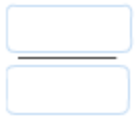# Problem: Construct the following SI derived unit, the coulomb, and define the faraday. A coulomb is a measure of _________  and has units of: 1  _____, _____ .A faraday is a constant and has a value of:  (include units). C        sA        96,485 CV        mole of electrons

###### FREE Expert Solution

Coulomb → SI Unit for electric charge; Unit is C or A•s

90% (23 ratings)###### Problem Details

Construct the following SI derived unit, the coulomb, and define the faraday.

A coulomb is a measure of _________  and has units of: 1  _____, _____ .

A faraday is a constant and has a value of:(include units).

C        s

A        96,485 C

V        mole of electrons